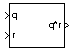# Quaternion Multiplication

Calculate product of two quaternions

• Library:
• Aerospace Blockset / Utilities / Math Operations

•## Description

The Quaternion Multiplication block calculates the product for two given quaternions. Aerospace Blockset™ uses quaternions that are defined using the scalar-first convention. For more information on the quaternion forms, see Algorithms.

## Ports

### Input

expand all

First quaternion, specified as a vector or vector of quaternions. A vector of quaternions has this form, where q and p are quaternions:

```[ q 0 , p 0 , ..., q 1 , p 1 , ... , q 2 , p 2 , ... , q 3 , p 3 , ...]```

Data Types: `double`

Second quaternion, specified as a vector or vector of quaternions. A vector of quaternions has this form, where s and r are quaternions:

```[ s 0 , r 0 , ..., s 1 , r 1 , ... , s 2 , r 2 , ... , s 3 , r 3 , ...]```

Data Types: `double`

### Output

expand all

Product of two quaternions, output as a vector or vector of quaternion products.

Data Types: `double`

## Algorithms

This block uses quaternions of the form of

`$q={q}_{0}+i{q}_{1}+j{q}_{2}+k{q}_{3}$`

and

`$r={r}_{0}+i{r}_{1}+j{r}_{2}+k{r}_{3}.$`

The quaternion product has the form of

`$t=q×r={t}_{0}+i{t}_{1}+j{t}_{2}+k{t}_{3},$`

where

`$\begin{array}{l}{t}_{0}=\left({r}_{0}{q}_{0}-{r}_{1}{q}_{1}-{r}_{2}{q}_{2}-{r}_{3}{q}_{3}\right)\\ {t}_{1}=\left({r}_{0}{q}_{1}+{r}_{1}{q}_{0}-{r}_{2}{q}_{3}+{r}_{3}{q}_{2}\right)\\ {t}_{2}=\left({r}_{0}{q}_{2}+{r}_{1}{q}_{3}+{r}_{2}{q}_{0}-{r}_{3}{q}_{1}\right)\\ {t}_{3}=\left({r}_{0}{q}_{3}-{r}_{1}{q}_{2}+{r}_{2}{q}_{1}+{r}_{3}{q}_{0}\right)\end{array}$`

 Stevens, Brian L., Frank L. Lewis. Aircraft Control and Simulation, Second Edition. Hoboken, NJ: Wiley–Interscience.